# Concise Selina Solutions for Class 9 Maths Chapter 18- Statistics

The Selina solutions for the questions given in Chapter 18, Statistics, of the Class 9 Selina textbooks are available here. In this chapter students learn about the topic of Statistics in detail. Students can easily score full marks in the exams by solving all the questions present in the Selina textbook.

The Class 9 Selina solutions are very easy to understand. These Class 9 Selina solutions maths cover all the exercise questions included in the book and are according to the syllabus prescribed by the ICSE or CISCE. Here, the PDF of the Class 9 maths Chapter 18 Selina solutions is available which can be downloaded as well as viewed online. Students can also avail these Selina solutions and download it for free to practice them offline as well.

### Download PDF of Selina Solutions for Class 9 Maths Chapter 18:-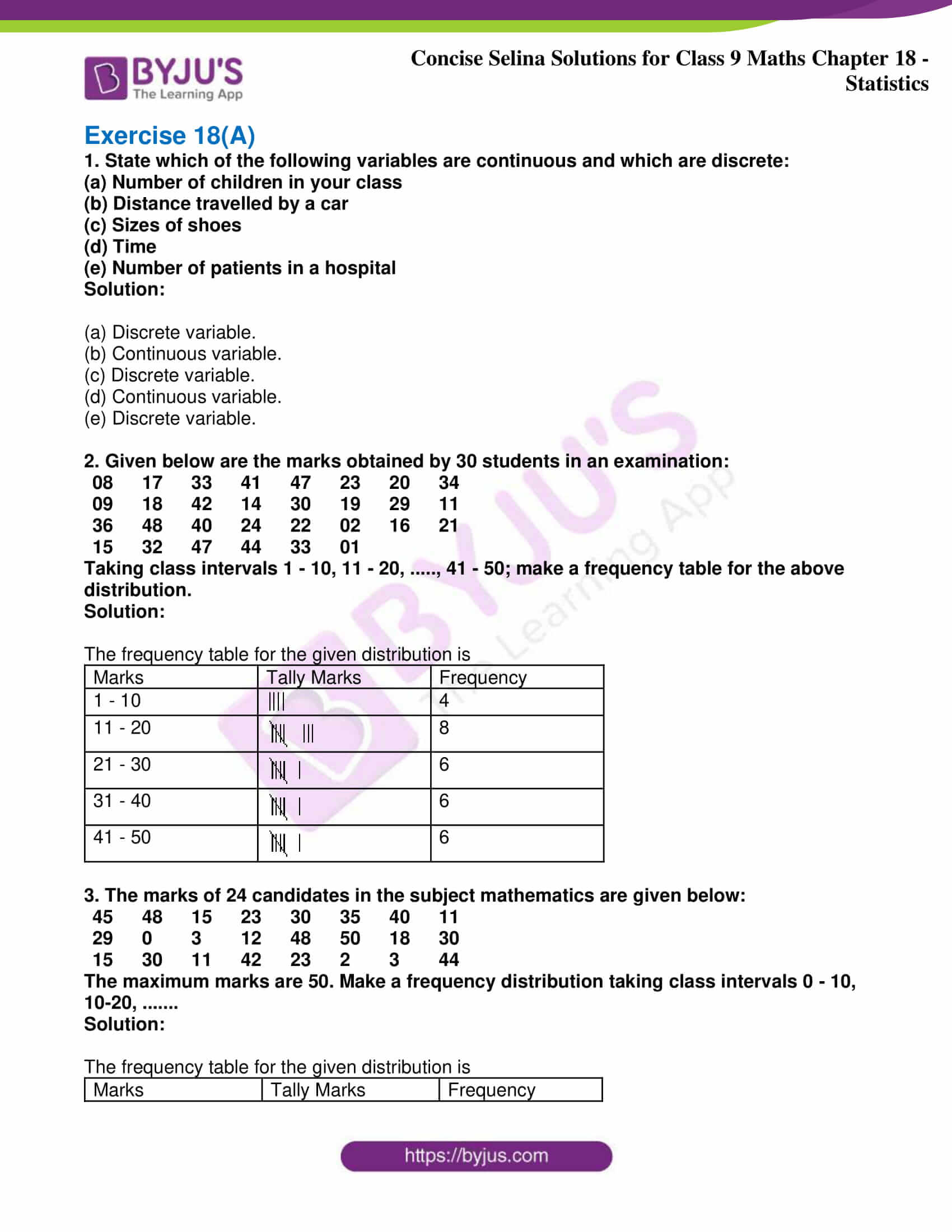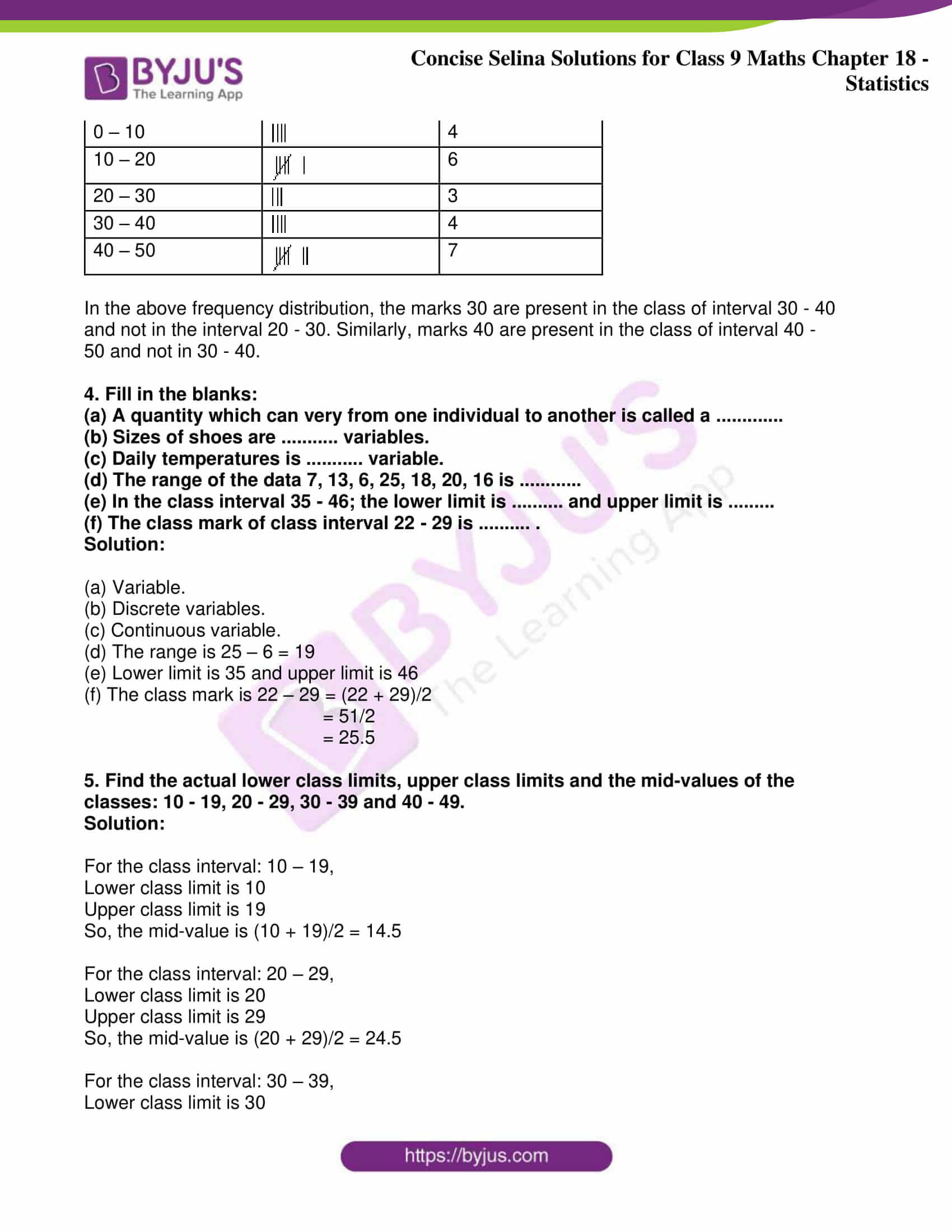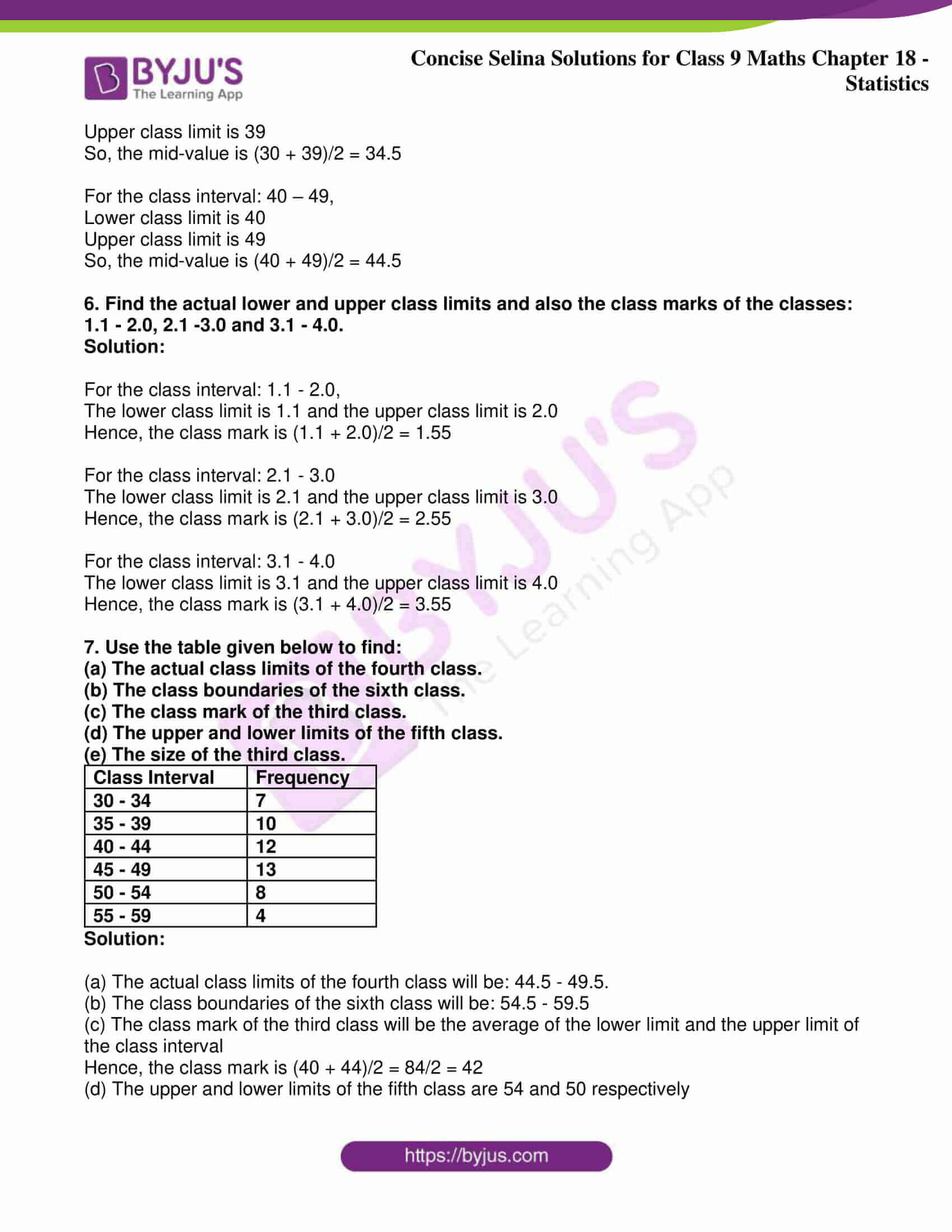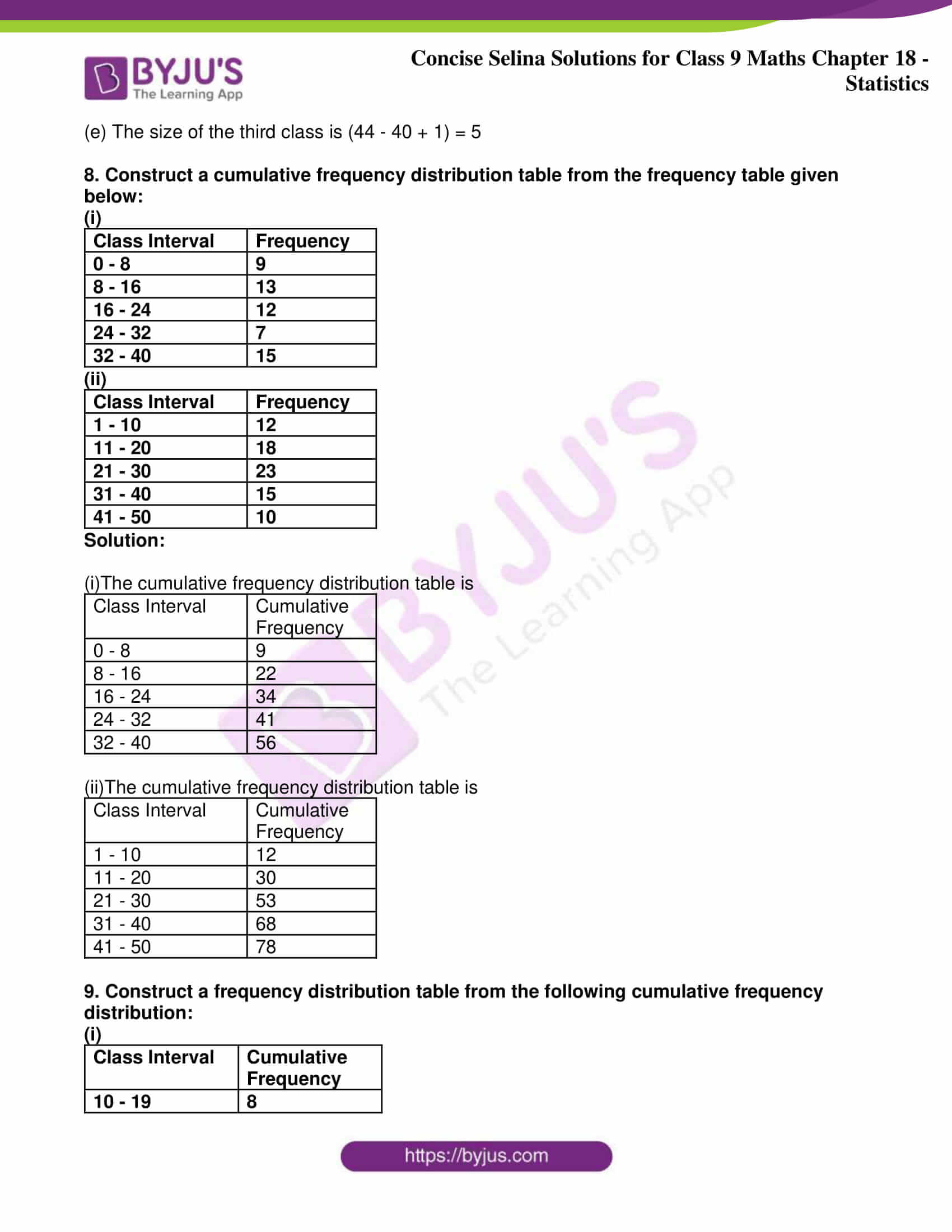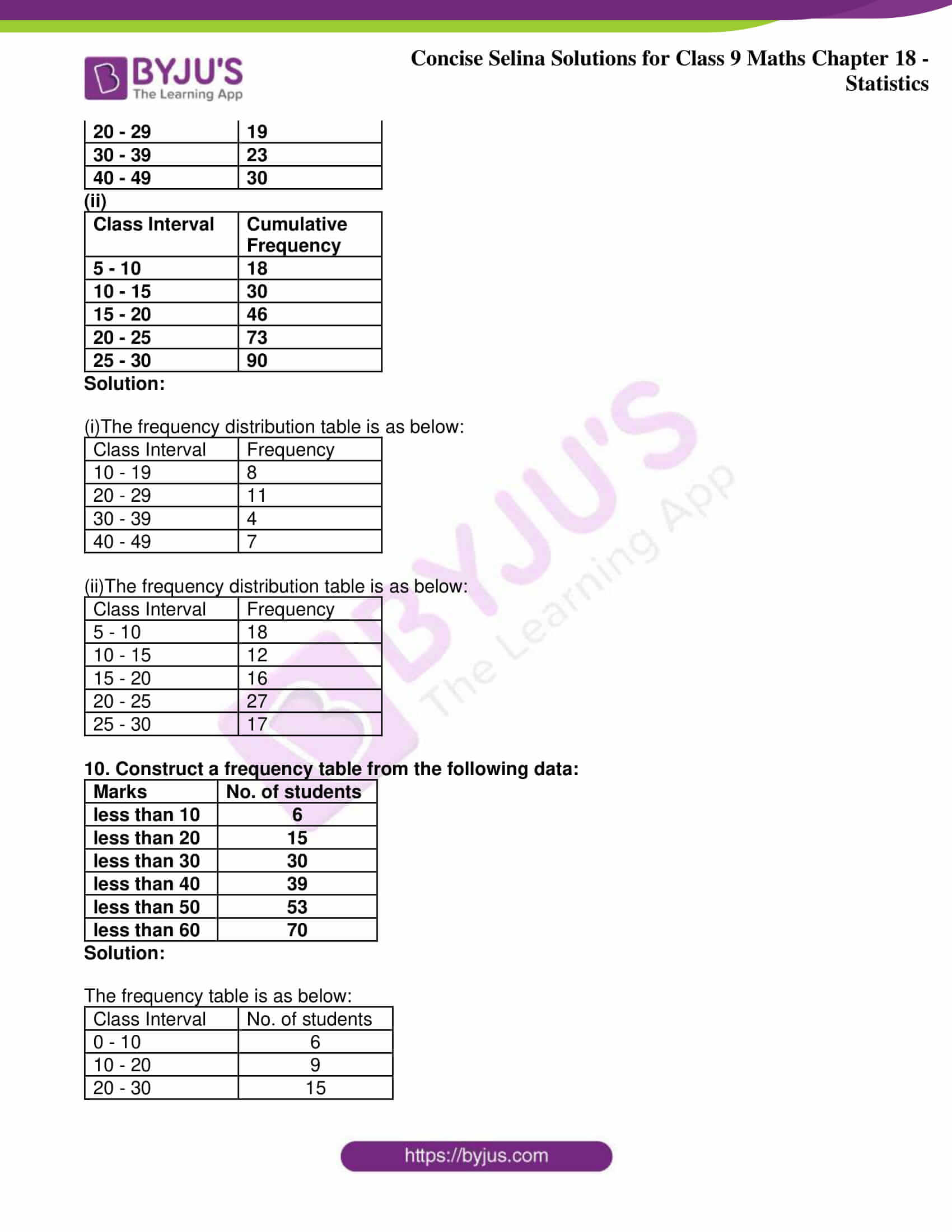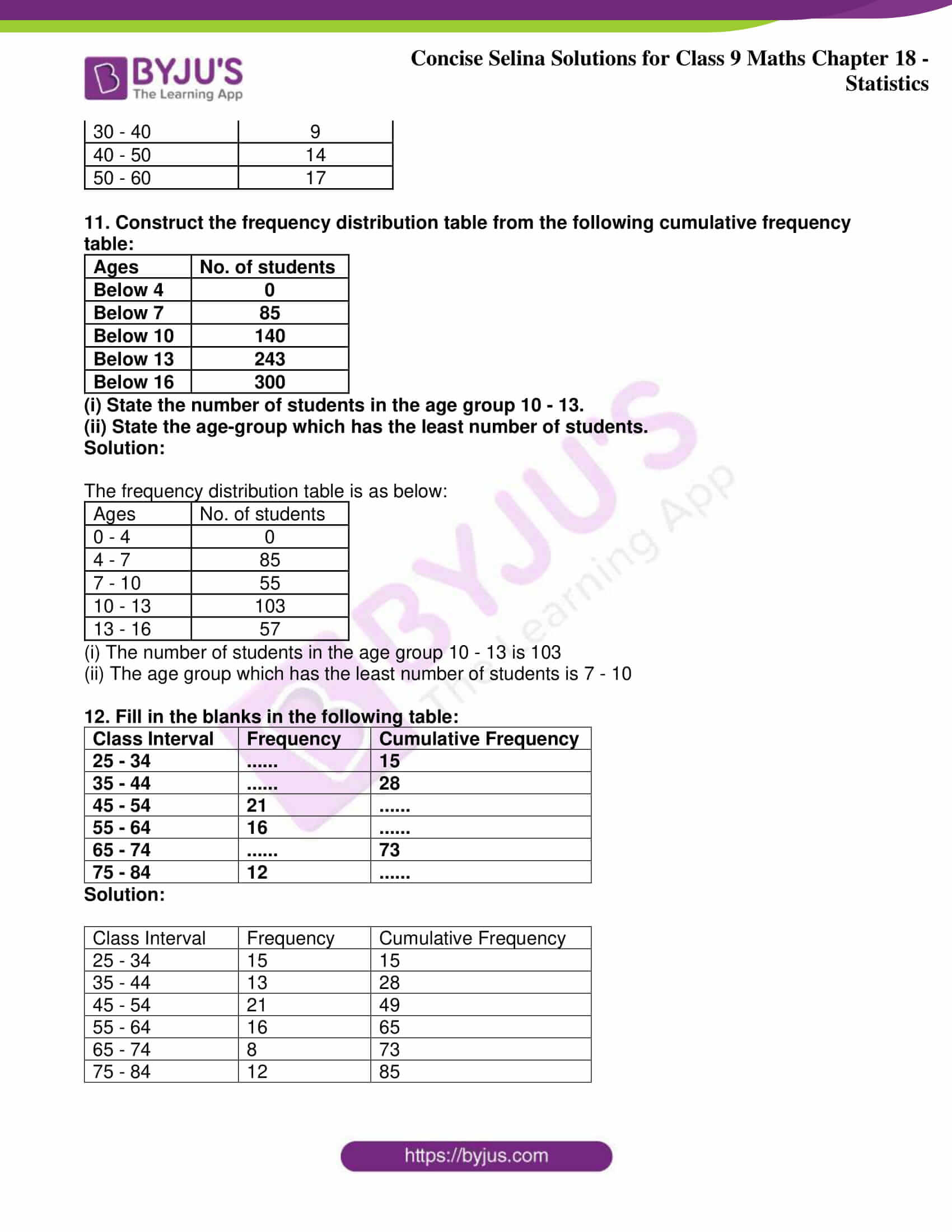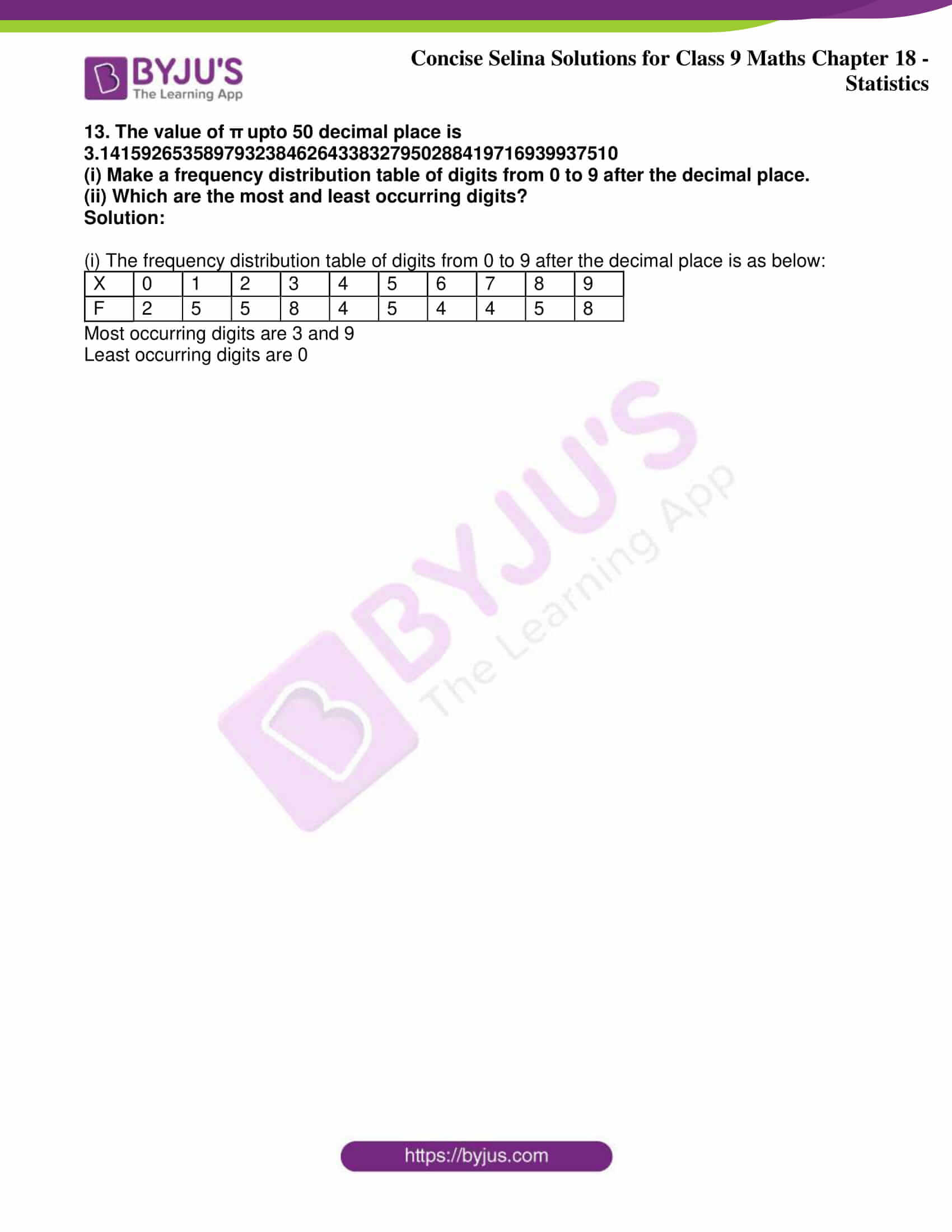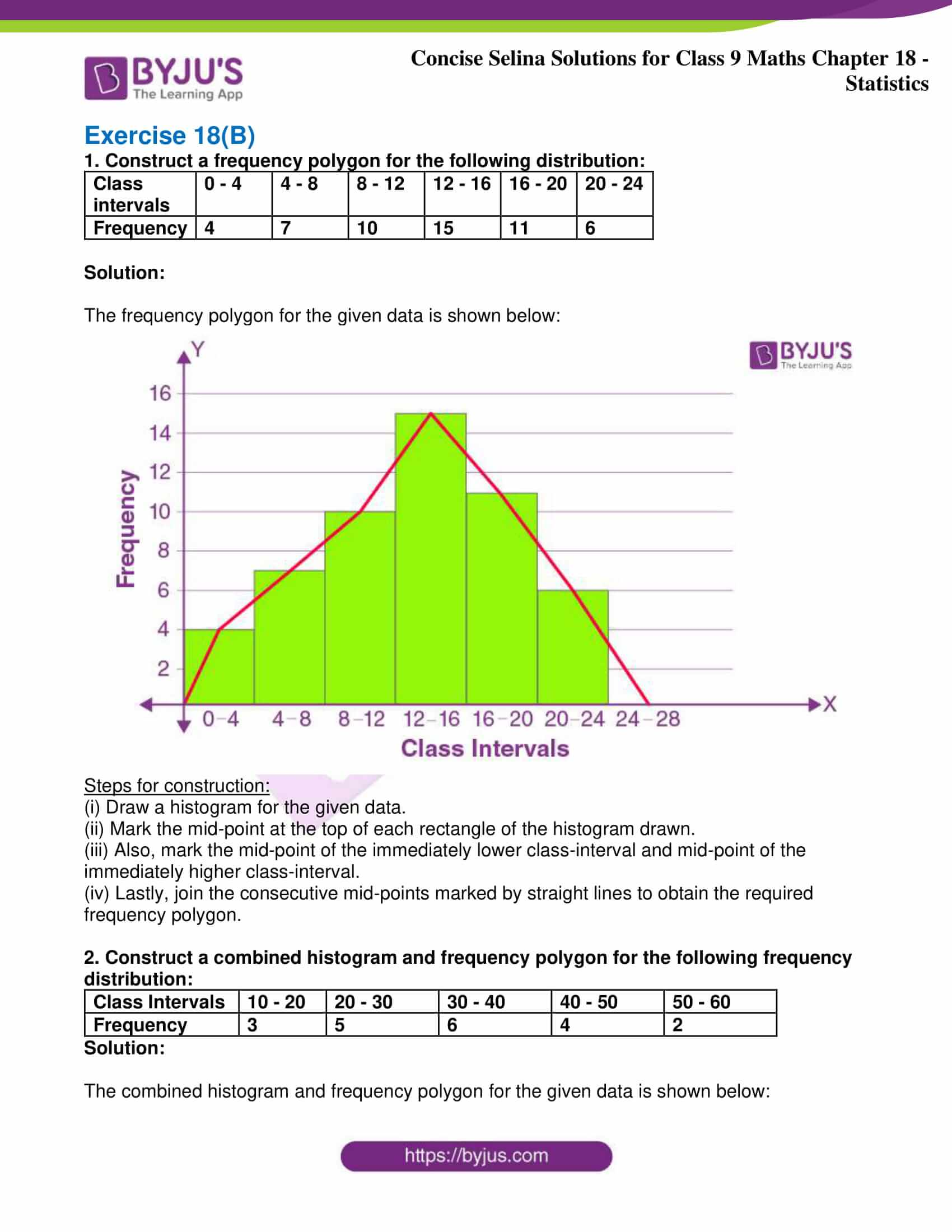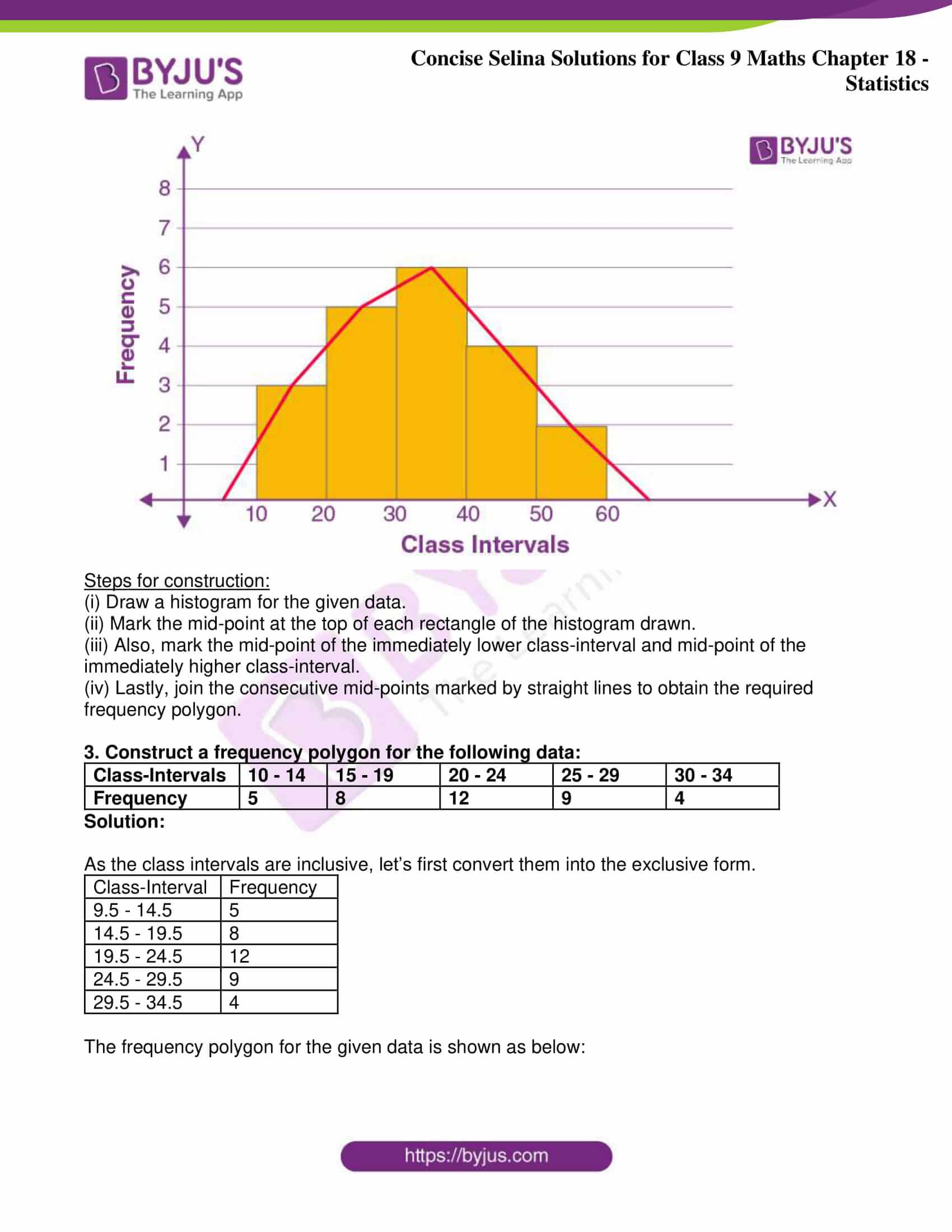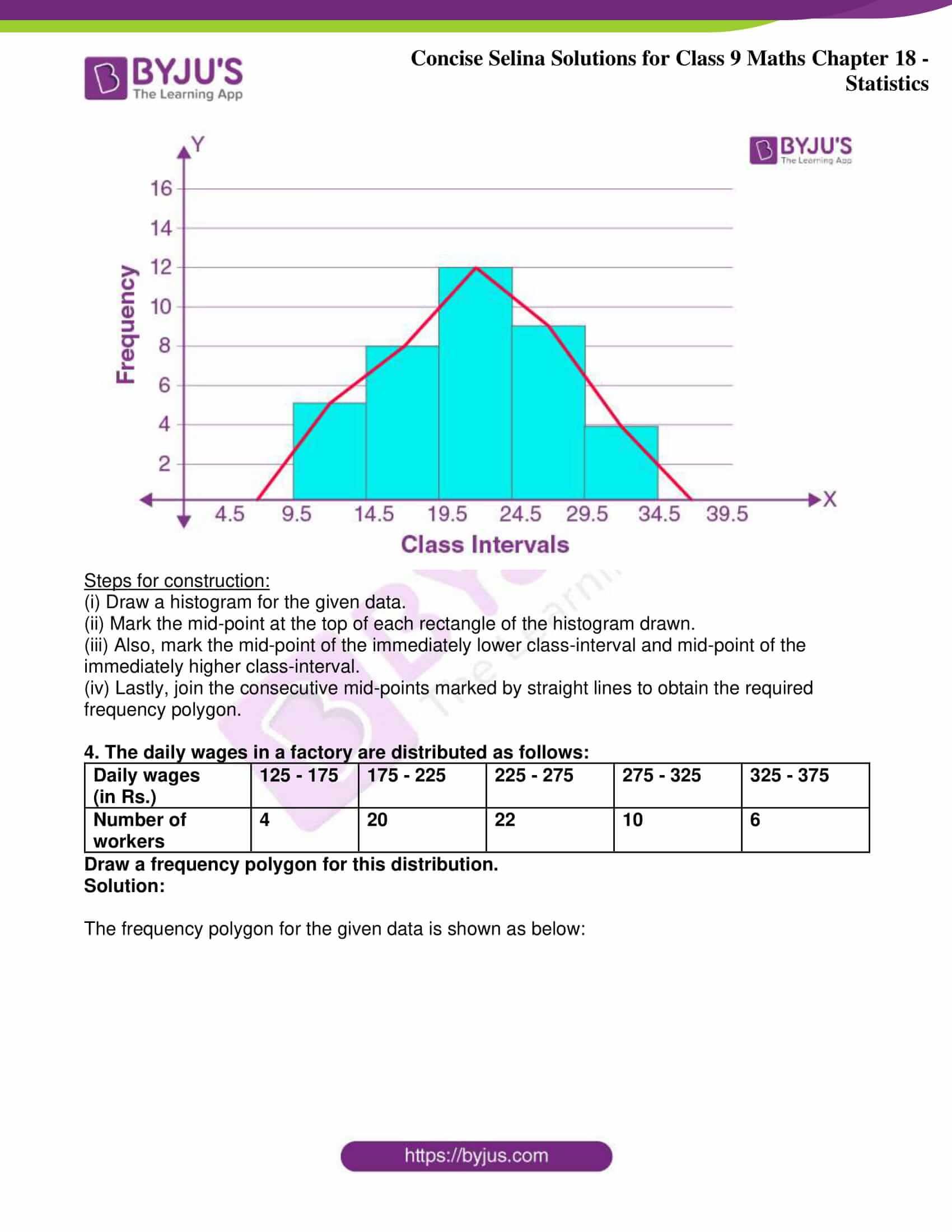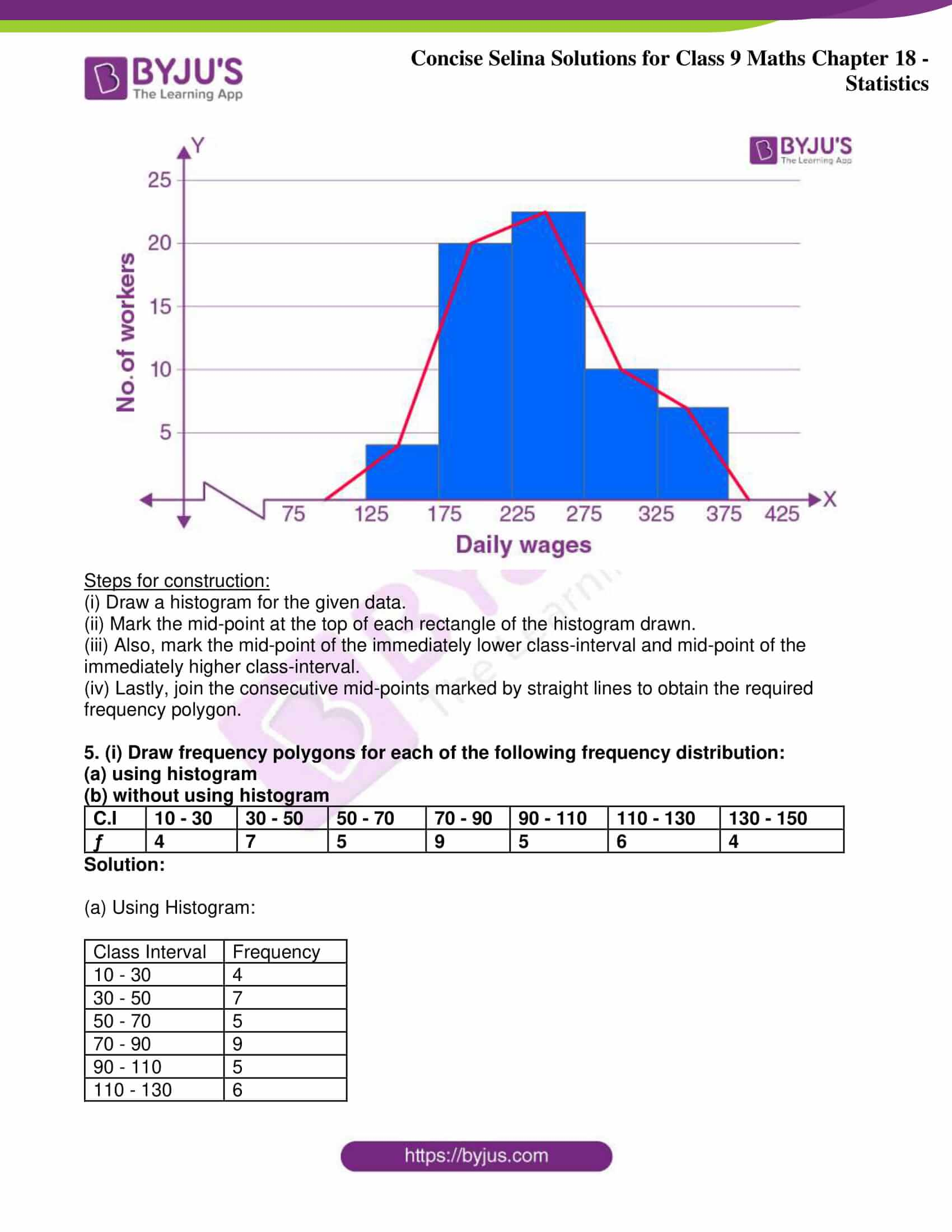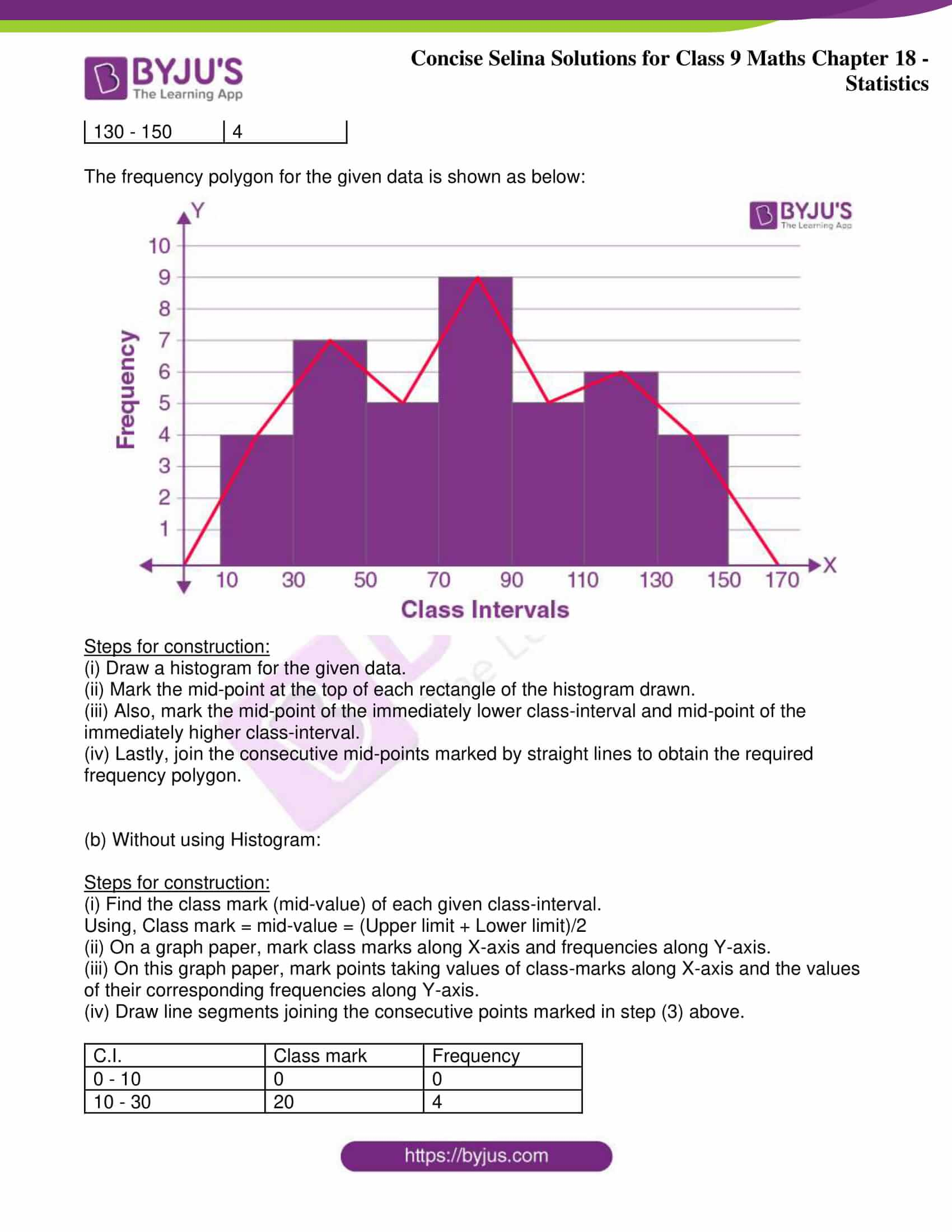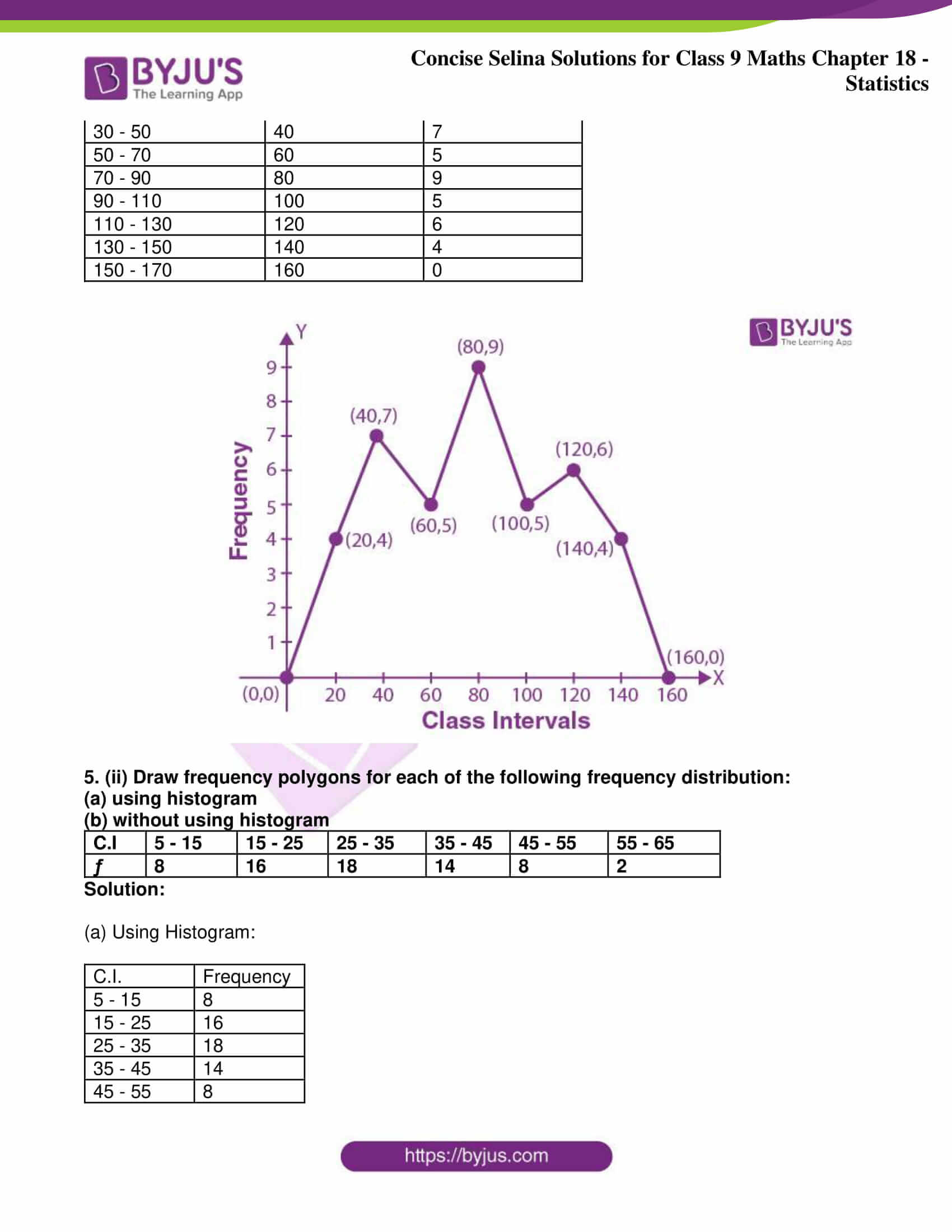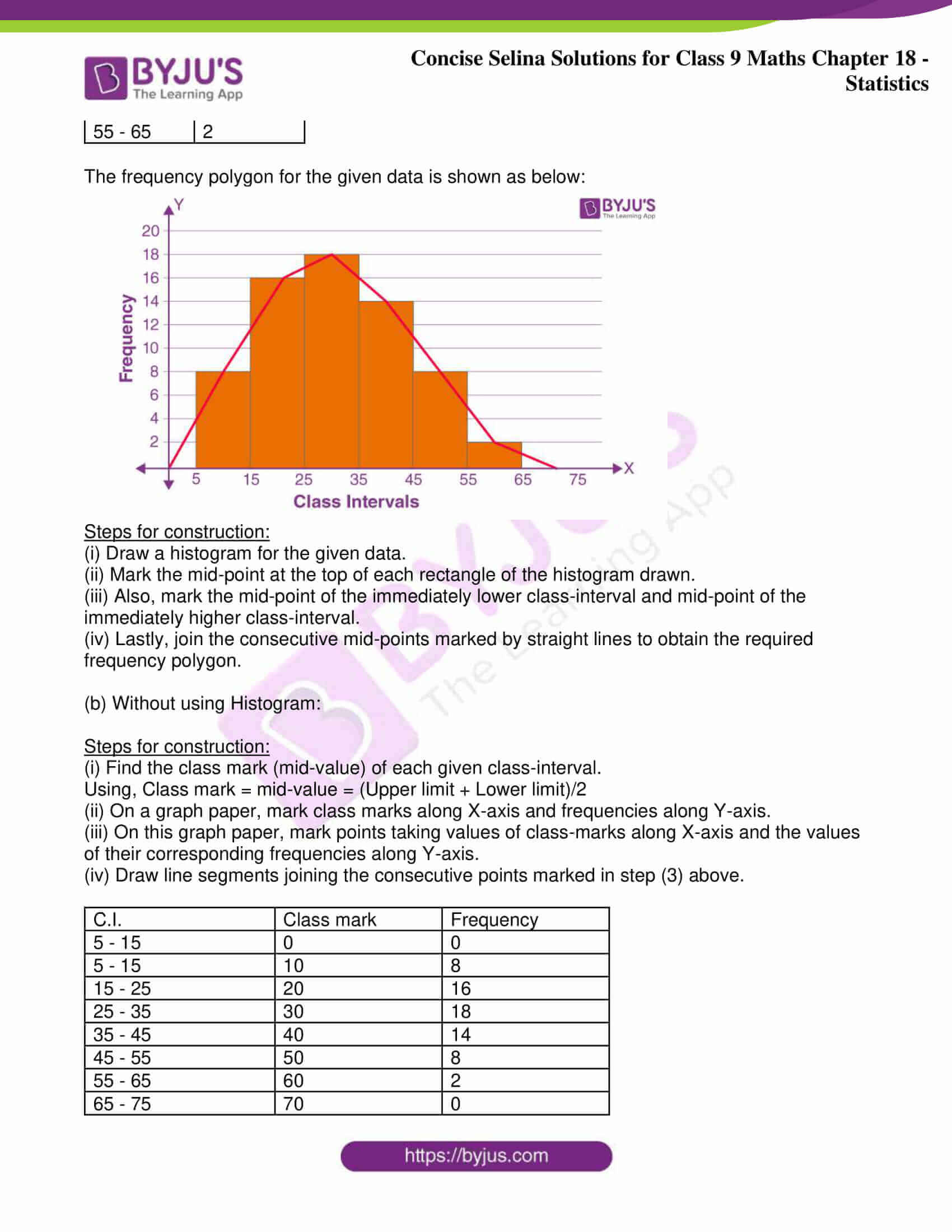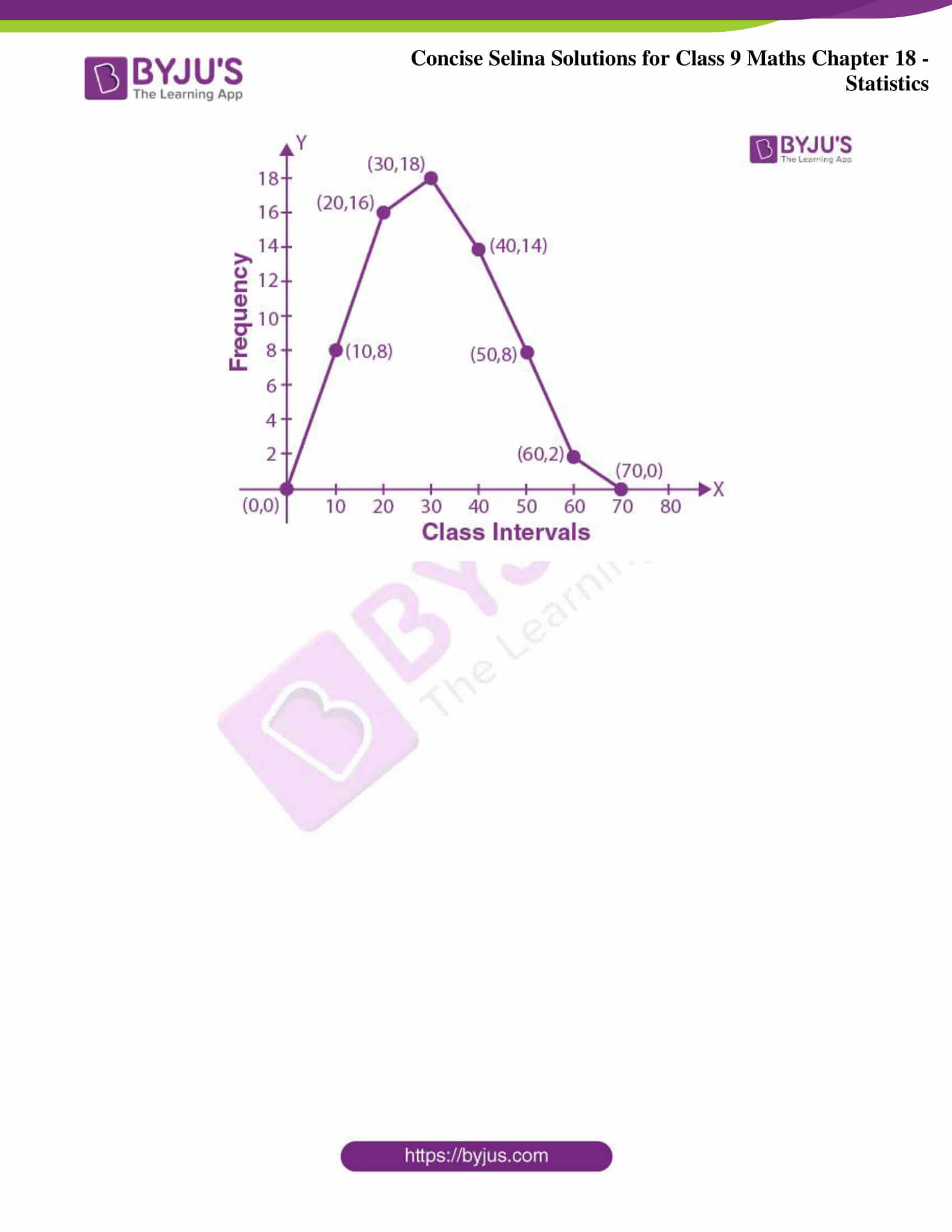Exercise 18(A)

1. State which of the following variables are continuous and which are discrete:

(a) Number of children in your class

(b) Distance travelled by a car

(c) Sizes of shoes

(d) Time

(e) Number of patients in a hospital

Solution:

(a) Discrete variable.

(b) Continuous variable.

(c) Discrete variable.

(d) Continuous variable.

(e) Discrete variable.

2. Given below are the marks obtained by 30 students in an examination:

 08 17 33 41 47 23 20 34 09 18 42 14 30 19 29 11 36 48 40 24 22 02 16 21 15 32 47 44 33 01

Taking class intervals 1 – 10, 11 – 20, ….., 41 – 50; make a frequency table for the above distribution.

Solution:

The frequency table for the given distribution is

 Marks Tally Marks Frequency 1 – 10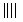4 11 – 20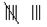8 21 – 30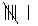6 31 – 40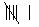6 41 – 50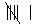6

3. The marks of 24 candidates in the subject mathematics are given below:

 45 48 15 23 30 35 40 11 29 0 3 12 48 50 18 30 15 30 11 42 23 2 3 44

The maximum marks are 50. Make a frequency distribution taking class intervals 0 – 10, 10-20, …….

Solution:

The frequency table for the given distribution is

 Marks Tally Marks Frequency 0 – 10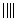4 10 – 20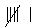6 20 – 30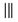3 30 – 40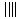4 40 – 50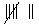7

In the above frequency distribution, the marks 30 are present in the class of interval 30 – 40 and not in the interval 20 – 30. Similarly, marks 40 are present in the class of interval 40 – 50 and not in 30 – 40.

4. Fill in the blanks:

(a) A quantity which can very from one individual to another is called a ………….

(b) Sizes of shoes are ……….. variables.

(c) Daily temperatures is ……….. variable.

(d) The range of the data 7, 13, 6, 25, 18, 20, 16 is …………

(e) In the class interval 35 – 46; the lower limit is ………. and upper limit is ………

(f) The class mark of class interval 22 – 29 is ………. .

Solution:

(a) Variable.

(b) Discrete variables.

(c) Continuous variable.

(d) The range is 25 – 6 = 19

(e) Lower limit is 35 and upper limit is 46

(f) The class mark is 22 – 29 = (22 + 29)/2

= 51/2

= 25.5

5. Find the actual lower class limits, upper class limits and the mid-values of the classes: 10 – 19, 20 – 29, 30 – 39 and 40 – 49.

Solution:

For the class interval: 10 – 19,

Lower class limit is 10

Upper class limit is 19

So, the mid-value is (10 + 19)/2 = 14.5

For the class interval: 20 – 29,

Lower class limit is 20

Upper class limit is 29

So, the mid-value is (20 + 29)/2 = 24.5

For the class interval: 30 – 39,

Lower class limit is 30

Upper class limit is 39

So, the mid-value is (30 + 39)/2 = 34.5

For the class interval: 40 – 49,

Lower class limit is 40

Upper class limit is 49

So, the mid-value is (40 + 49)/2 = 44.5

6. Find the actual lower and upper class limits and also the class marks of the classes:

1.1 – 2.0, 2.1 -3.0 and 3.1 – 4.0.

Solution:

For the class interval: 1.1 – 2.0,

The lower class limit is 1.1 and the upper class limit is 2.0

Hence, the class mark is (1.1 + 2.0)/2 = 1.55

For the class interval: 2.1 – 3.0

The lower class limit is 2.1 and the upper class limit is 3.0

Hence, the class mark is (2.1 + 3.0)/2 = 2.55

For the class interval: 3.1 – 4.0

The lower class limit is 3.1 and the upper class limit is 4.0

Hence, the class mark is (3.1 + 4.0)/2 = 3.55

7. Use the table given below to find:

(a) The actual class limits of the fourth class.

(b) The class boundaries of the sixth class.

(c) The class mark of the third class.

(d) The upper and lower limits of the fifth class.

(e) The size of the third class.

 Class Interval Frequency 30 – 34 7 35 – 39 10 40 – 44 12 45 – 49 13 50 – 54 8 55 – 59 4

Solution:

(a) The actual class limits of the fourth class will be: 44.5 – 49.5.

(b) The class boundaries of the sixth class will be: 54.5 – 59.5

(c) The class mark of the third class will be the average of the lower limit and the upper limit of the class interval

Hence, the class mark is (40 + 44)/2 = 84/2 = 42

(d) The upper and lower limits of the fifth class are 54 and 50 respectively

(e) The size of the third class is (44 – 40 + 1) = 5

8. Construct a cumulative frequency distribution table from the frequency table given below:

(i)

 Class Interval Frequency 0 – 8 9 8 – 16 13 16 – 24 12 24 – 32 7 32 – 40 15

(ii)

 Class Interval Frequency 1 – 10 12 11 – 20 18 21 – 30 23 31 – 40 15 41 – 50 10

Solution:

(i)The cumulative frequency distribution table is

 Class Interval Cumulative Frequency 0 – 8 9 8 – 16 22 16 – 24 34 24 – 32 41 32 – 40 56

(ii)The cumulative frequency distribution table is

 Class Interval Cumulative Frequency 1 – 10 12 11 – 20 30 21 – 30 53 31 – 40 68 41 – 50 78

9. Construct a frequency distribution table from the following cumulative frequency distribution:

(i)

 Class Interval Cumulative Frequency 10 – 19 8 20 – 29 19 30 – 39 23 40 – 49 30

(ii)

 Class Interval Cumulative Frequency 5 – 10 18 10 – 15 30 15 – 20 46 20 – 25 73 25 – 30 90

Solution:

(i)The frequency distribution table is as below:

 Class Interval Frequency 10 – 19 8 20 – 29 11 30 – 39 4 40 – 49 7

(ii)The frequency distribution table is as below:

 Class Interval Frequency 5 – 10 18 10 – 15 12 15 – 20 16 20 – 25 27 25 – 30 17

10. Construct a frequency table from the following data:

 Marks No. of students less than 10 6 less than 20 15 less than 30 30 less than 40 39 less than 50 53 less than 60 70

Solution:

The frequency table is as below:

 Class Interval No. of students 0 – 10 6 10 – 20 9 20 – 30 15 30 – 40 9 40 – 50 14 50 – 60 17

11. Construct the frequency distribution table from the following cumulative frequency table:

 Ages No. of students Below 4 0 Below 7 85 Below 10 140 Below 13 243 Below 16 300

(i) State the number of students in the age group 10 – 13.

(ii) State the age-group which has the least number of students.

Solution:

The frequency distribution table is as below:

 Ages No. of students 0 – 4 0 4 – 7 85 7 – 10 55 10 – 13 103 13 – 16 57

(i) The number of students in the age group 10 – 13 is 103

(ii) The age group which has the least number of students is 7 – 10

12. Fill in the blanks in the following table:

 Class Interval Frequency Cumulative Frequency 25 – 34 …… 15 35 – 44 …… 28 45 – 54 21 …… 55 – 64 16 …… 65 – 74 …… 73 75 – 84 12 ……

Solution:

 Class Interval Frequency Cumulative Frequency 25 – 34 15 15 35 – 44 13 28 45 – 54 21 49 55 – 64 16 65 65 – 74 8 73 75 – 84 12 85

13. The value of π upto 50 decimal place is

3.14159265358979323846264338327950288419716939937510

(i) Make a frequency distribution table of digits from 0 to 9 after the decimal place.

(ii) Which are the most and least occurring digits?

Solution:

(i) The frequency distribution table of digits from 0 to 9 after the decimal place is as below:

 X 0 1 2 3 4 5 6 7 8 9 F 2 5 5 8 4 5 4 4 5 8

Most occurring digits are 3 and 9

Least occurring digits are 0

Exercise 18(B)

1. Construct a frequency polygon for the following distribution:

 Class intervals 0 – 4 4 – 8 8 – 12 12 – 16 16 – 20 20 – 24 Frequency 4 7 10 15 11 6

Solution:

The frequency polygon for the given data is shown below: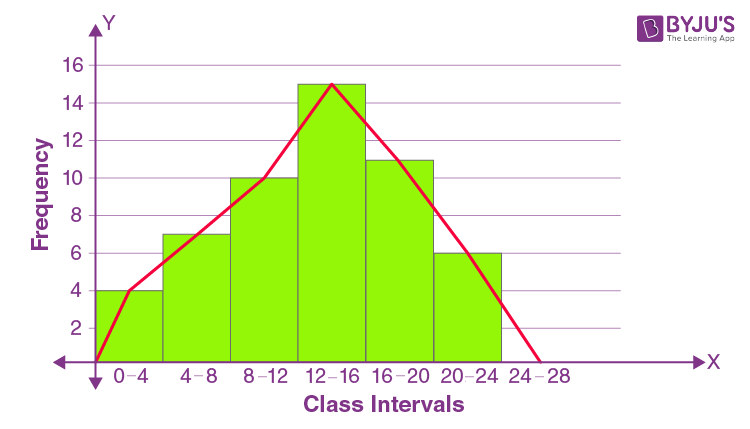Steps for construction:

(i) Draw a histogram for the given data.

(ii) Mark the mid-point at the top of each rectangle of the histogram drawn.

(iii) Also, mark the mid-point of the immediately lower class-interval and mid-point of the immediately higher class-interval.

(iv) Lastly, join the consecutive mid-points marked by straight lines to obtain the required frequency polygon.

2. Construct a combined histogram and frequency polygon for the following frequency distribution:

 Class Intervals 10 – 20 20 – 30 30 – 40 40 – 50 50 – 60 Frequency 3 5 6 4 2

Solution:

The combined histogram and frequency polygon for the given data is shown below:Steps for construction:

(i) Draw a histogram for the given data.

(ii) Mark the mid-point at the top of each rectangle of the histogram drawn.

(iii) Also, mark the mid-point of the immediately lower class-interval and mid-point of the immediately higher class-interval.

(iv) Lastly, join the consecutive mid-points marked by straight lines to obtain the required frequency polygon.

3. Construct a frequency polygon for the following data:

 Class-Intervals 10 – 14 15 – 19 20 – 24 25 – 29 30 – 34 Frequency 5 8 12 9 4

Solution:

As the class intervals are inclusive, let’s first convert them into the exclusive form.

 Class-Interval Frequency 9.5 – 14.5 5 14.5 – 19.5 8 19.5 – 24.5 12 24.5 – 29.5 9 29.5 – 34.5 4

The frequency polygon for the given data is shown as below: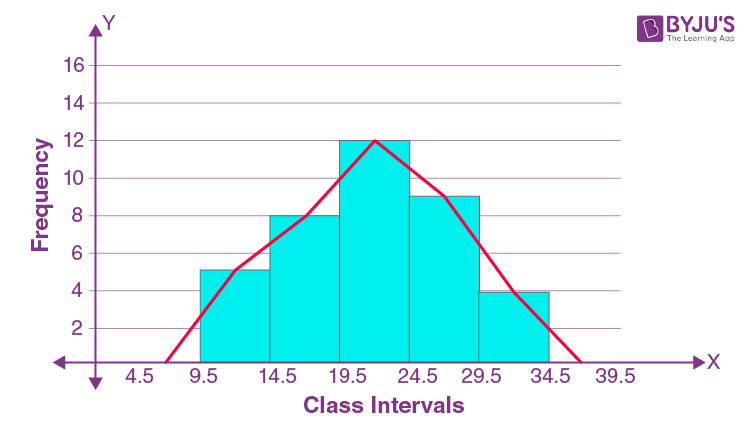Steps for construction:

(i) Draw a histogram for the given data.

(ii) Mark the mid-point at the top of each rectangle of the histogram drawn.

(iii) Also, mark the mid-point of the immediately lower class-interval and mid-point of the immediately higher class-interval.

(iv) Lastly, join the consecutive mid-points marked by straight lines to obtain the required frequency polygon.

4. The daily wages in a factory are distributed as follows:

 Daily wages (in Rs.) 125 – 175 175 – 225 225 – 275 275 – 325 325 – 375 Number of workers 4 20 22 10 6

Draw a frequency polygon for this distribution.

Solution:

The frequency polygon for the given data is shown as below: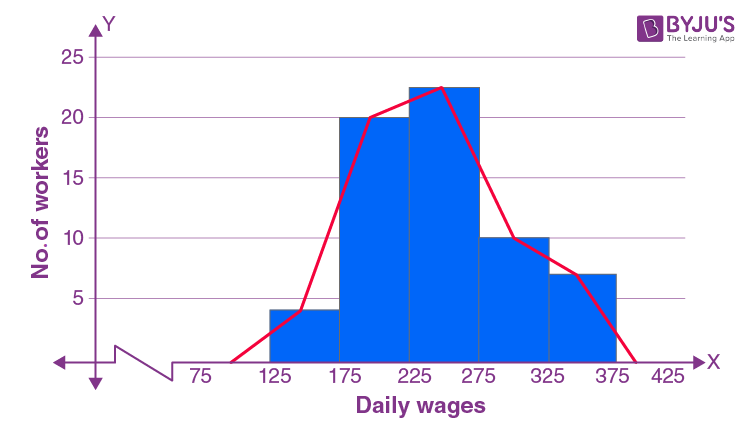Steps for construction:

(i) Draw a histogram for the given data.

(ii) Mark the mid-point at the top of each rectangle of the histogram drawn.

(iii) Also, mark the mid-point of the immediately lower class-interval and mid-point of the immediately higher class-interval.

(iv) Lastly, join the consecutive mid-points marked by straight lines to obtain the required frequency polygon.

5. (i) Draw frequency polygons for each of the following frequency distribution:

(a) using histogram

(b) without using histogram

 C.I 10 – 30 30 – 50 50 – 70 70 – 90 90 – 110 110 – 130 130 – 150 ƒ 4 7 5 9 5 6 4

Solution:

(a) Using Histogram:

 Class Interval Frequency 10 – 30 4 30 – 50 7 50 – 70 5 70 – 90 9 90 – 110 5 110 – 130 6 130 – 150 4

The frequency polygon for the given data is shown as below: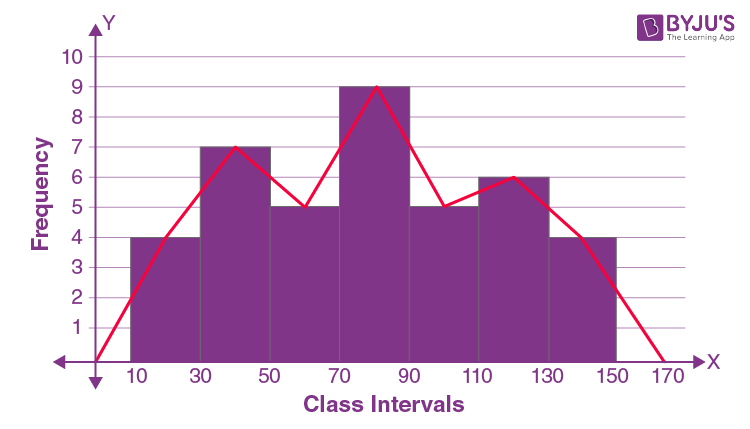Steps for construction:

(i) Draw a histogram for the given data.

(ii) Mark the mid-point at the top of each rectangle of the histogram drawn.

(iii) Also, mark the mid-point of the immediately lower class-interval and mid-point of the immediately higher class-interval.

(iv) Lastly, join the consecutive mid-points marked by straight lines to obtain the required frequency polygon.

(b) Without using Histogram:

Steps for construction:

(i) Find the class mark (mid-value) of each given class-interval.

Using, Class mark = mid-value = (Upper limit + Lower limit)/2

(ii) On a graph paper, mark class marks along X-axis and frequencies along Y-axis.

(iii) On this graph paper, mark points taking values of class-marks along X-axis and the values of their corresponding frequencies along Y-axis.

(iv) Draw line segments joining the consecutive points marked in step (3) above.

 C.I. Class mark Frequency 0 – 10 0 0 10 – 30 20 4 30 – 50 40 7 50 – 70 60 5 70 – 90 80 9 90 – 110 100 5 110 – 130 120 6 130 – 150 140 4 150 – 170 160 0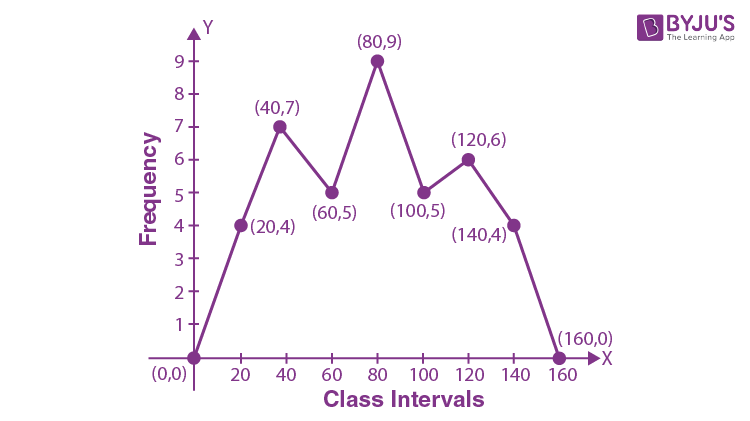5. (ii) Draw frequency polygons for each of the following frequency distribution:

(a) using histogram

(b) without using histogram

 C.I 5 – 15 15 – 25 25 – 35 35 – 45 45 – 55 55 – 65 ƒ 8 16 18 14 8 2

Solution:

(a) Using Histogram:

 C.I. Frequency 5 – 15 8 15 – 25 16 25 – 35 18 35 – 45 14 45 – 55 8 55 – 65 2

The frequency polygon for the given data is shown as below: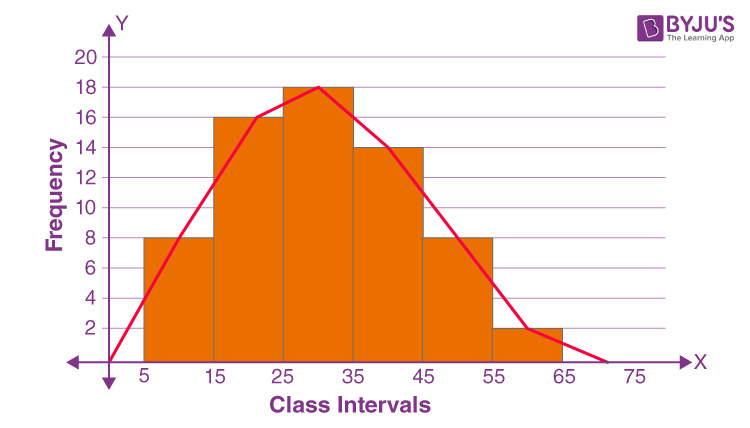Steps for construction:

(i) Draw a histogram for the given data.

(ii) Mark the mid-point at the top of each rectangle of the histogram drawn.

(iii) Also, mark the mid-point of the immediately lower class-interval and mid-point of the immediately higher class-interval.

(iv) Lastly, join the consecutive mid-points marked by straight lines to obtain the required frequency polygon.

(b) Without using Histogram:

Steps for construction:

(i) Find the class mark (mid-value) of each given class-interval.

Using, Class mark = mid-value = (Upper limit + Lower limit)/2

(ii) On a graph paper, mark class marks along X-axis and frequencies along Y-axis.

(iii) On this graph paper, mark points taking values of class-marks along X-axis and the values of their corresponding frequencies along Y-axis.

(iv) Draw line segments joining the consecutive points marked in step (3) above.

 C.I. Class mark Frequency 5 – 15 0 0 5 – 15 10 8 15 – 25 20 16 25 – 35 30 18 35 – 45 40 14 45 – 55 50 8 55 – 65 60 2 65 – 75 70 0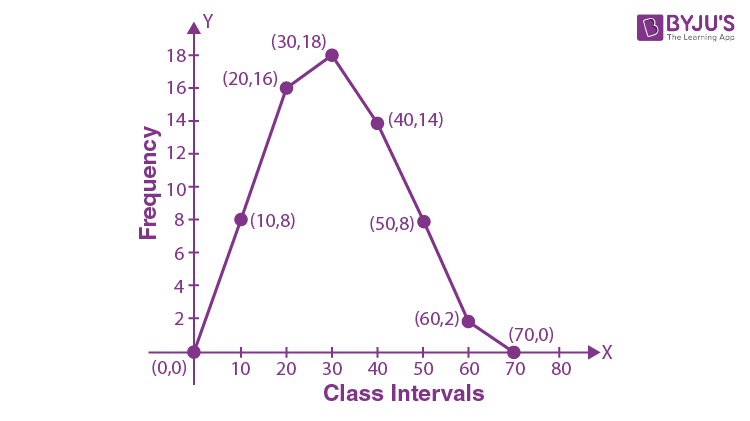## Selina Solutions for Class 9 Maths Chapter 18- Statistics

The Chapter 18, Statistics, contains 2 exercises and the Solutions given here contains the answers for all the questions present in these exercises. Let us have a look at some of the topics that are being discussed in this chapter.

18.1 Introduction

18.2 Variable

18.3 Tabulation of data

18.4 Frequency

18.5 Frequency distribution table

18.6 Types of frequency distributions

18.7 Class intervals and class limits

18.8 Cumulative frequency and cumulative frequency table

18.9 Graphical representation of data

18.10 Graphical representation of continuous frequency distribution

## Selina Solutions for Class 9 Maths Chapter 18- Statistics

Statistics is a branch of Mathematics that is concerned with how information is collected, organised, presented, summarised and then analysed so the conclusion may be drawn from the information. Read and learn the Chapter 18 of Selina textbook to get to know more about Statistics. Learn the Selina Solutions for Class 9 effectively to attain excellent result in the examination.